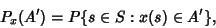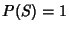## Distribution

The distribution of a variable is a description of the relative numbers of times each possible outcome will occur in a number of trials. The function describing the distribution is called the Probability Function, and the function describing the probability that a given value or any value smaller than it will occur is called the Distribution Function.

Formally, a distribution can be defined as a normalized Measure, and the distribution of a Random Variableis the Measureondefined by settingwhereis a Probability Space,is a Measurable Space, anda Measure onwith.

See also Continuous Distribution, Discrete Distribution, Distribution Function, Measurable Space, Measure, Probability, Probability Density Function, Random Variable, Statistics

References

Doob, J. L. The Development of Rigor in Mathematical Probability (1900-1950).'' Amer. Math. Monthly 103, 586-595, 1996.